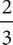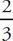# SAT Math Multiple Choice Question 90: Answer and Explanation

### Test Information

Question: 90

15. Bailey's Boutique Clothing is having a 20% off sale during which shirts cost \$30.00 and pants cost \$60.00. On the day of the sale, Bailey's sellsa total of 60 shirts and pants and earned a total of \$2,250. On a regular day, Bailey's sellsthe number of shirts and pants sold during the sale and earns a total of \$1,875. Solving which of the following systems of equations yields the number of shirts, s, and the number of pants, p, sold during a regular day?

• A. s + p = 40
37.5s + 75p = 1,875
• B. s + p = 40
30s + 60p = 2,250
• C. s + p = 60
30s + 60p = 2,250
• D. s + p = 2,250
30s + 60p = 60

A Start with the easier equation and use Process of Elimination. The easier equation is related to the total number of shirts and pants, s + p, sold on a regular day. The question states that on a regular day Bailey's sellsthe number of pants and shirts sold during a sale.(60) = 40. Therefore, one of the equations in the correct answer should be s + p = 40. Eliminate (C) and (D) since neither of these answers include this equation. The other equation is related to themoney Bailey's earns on a regular day. According to the question, Bailey's earns a total of \$1,875 on a regular day, so the equation must equal \$1,875. Eliminate (B) because the total in the money equation is incorrect. The correct answer is (A).